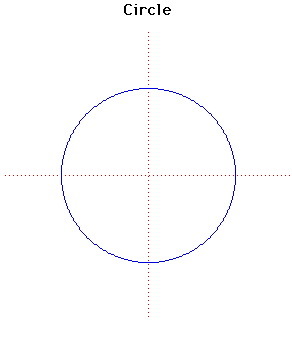# CircleCartesian equation:
x2 + y2 = a2
or parametrically:
x = a cos(t), y = a sin(t)
Polar equation:
r = a

Click below to see one of the Associated curves.

Click THIS LINK to experiment interactively with this curve and its associated curves.

The study of the circle goes back beyond recorded history. The invention of the wheel is a fundamental discovery of properties of a circle.

The greeks considered the Egyptians as the inventors of geometry. The scribe Ahmes, the author of the Rhind papyrus, gives a rule for determining the area of a circle which corresponds to π = 256/81 or approximately 3.16.

The first theorems relating to circles are attributed to Thales around 650 BC. Book III of Euclid's Elements deals with properties of circles and problems of inscribing and escribing polygons.

One of the problems of Greek mathematics was the problem of finding a square with the same area as a given circle. Several of the 'famous curves' in this stack were first studied in an attempt to solve this problem. Anaxagoras in 450 BC is the first recored mathematician to study this problem.

The problem of finding the area of a circle led to integration. For the circle with formula given above the area is πa2 and the length of the curve is 2πa.

The pedal of a circle is a cardioid if the pedal point is taken on the circumference and is a limacon if the pedal point is not on the circumference.

The caustic of a circle with radiant point on the circumference is a cardioid, while if the rays are parallel then the caustic is a nephroid.

Apollonius, in about 240 BC, showed effectively that the bipolar equation r = kr' represents a system of coaxial circles as k varies. In terms of bipolar equations mr2 + nr'2 = c2 represents a circle whose centre divides the line segment between the two fixed points of the system in the ratio n to m.

JOC/EFR/BS January 1997